• 但有时需要计算力在某个平面内的分量，即力在平面的投影，或者计算力垂直于某平面的分量，都可通过投影解决。 几何上，也经常涉及投影，如点到直线或平面的距离，此距离点到直线或平面的最短距离，求此距离可...
向量投影
力的正交分解就是投影，高中时一般向坐标轴投影，有时也需计算力在任意方向分量，即力在这个方向的投影，可通过内积计算。但有时需要计算力在某个平面内的分量，即力在平面内的投影，或者计算力垂直于某平面的分量，都可通过投影解决。
几何上，也经常涉及投影，如点到直线或平面的距离，此距离是点到直线或平面的最短距离，求此距离可通过投影解决。
再举个例子，为什么称为“投影”，投影就是物体在太阳光的照射下在地面形成的影子。当太阳光与地面垂直时是正投影，这就是线性代数中研究的投影。当物体与地面垂直时，影子长度（投影）为0。中国古时利用投影来计时，发明了日晷。希望读者根据物理学和几何学，获得投影的几何图像。
当转向高维空间时，投影不局限于低维子空间，可投向任意维度的子空间，所以需要代数方法，但理解需要几何图像。向量在子空间的投影，本质上是向量的正交分解，分解为两个向量：一个向量位于子空间，另一个位于其正交补空间。
子空间 $S_1$ 由无关组 $V = (\mathbf{v_1},\cdots,\mathbf{v_n})$ 张成，如何求任意向量 $\mathbf{v}$ 在子空间的投影向量呢？这是线性代数基本问题之一，也是理解线性方程的核心之一，必须十分重视。
投影向量位于子空间 $S_1$ 内，故其可表示为生成向量的线性组合，这是解决问题的关键一步，令投影向量为 $\mathbf{v}^{\bot} = \alpha_1\mathbf{v_1}+\cdots+\alpha_n\mathbf{v_n}$ ，只要算出表示系数组就可得到投影向量。另一正交分量 $\mathbf{v}^{-} = \mathbf{v}-\mathbf{v}^{\bot}$ 位于正交补空间 $S_1^{\bot}$ ，垂直子空间 $S_1$ 内任意向量。故两个分向量垂直，内积为零！这是关键第二步。
$0 = (\mathbf{v}^{\bot}, \mathbf{v}^{-}) = (\mathbf{v}^{\bot}, \mathbf{v}-\mathbf{v}^{\bot}) = (\mathbf{v}^{\bot}, \mathbf{v}) - (\mathbf{v}^{\bot}, \mathbf{v}^{\bot})\\ 得：(\mathbf{v}^{\bot}, \mathbf{v}) = (\mathbf{v}^{\bot}, \mathbf{v}^{\bot}) \\ 得：(\alpha_1\mathbf{v_1}+\cdots+\alpha_n\mathbf{v_n}, \mathbf{v}) =(\alpha_1\mathbf{v_1}+\cdots+\alpha_n\mathbf{v_n},\alpha_1\mathbf{v_1}+\cdots+\alpha_n\mathbf{v_n})=\sum_{ij}\alpha_i\alpha_j(\mathbf{v_i},\mathbf{v_j})$
根据正交分解的唯一性，$\mathbf{v}^{\bot}$ 是唯一的，根据无关组表示向量的唯一性，表示系数组存在且唯一。无关组是任意向量时，利用向量理论很难表示出表示系数，必须用矩阵理论才能方便表示。
无关组是标准正交基时，却很容易求出表示系数。 根据标准正交基性质： $(\mathbf{v_i},\mathbf{v_j})=0,\forall i\ne j; \quad =1 ，\forall i= j$ 。代入上式，
$(\alpha_1\mathbf{v_1}+\cdots+\alpha_n\mathbf{v_n}, \mathbf{v}) = \alpha_1(\mathbf{v_1}, \mathbf{v})+\cdots+\alpha_n(\mathbf{v_n}, \mathbf{v}) = \alpha_1^2+\cdots+\alpha_n^2$
显然当 $\alpha_i = (\mathbf{v_i}, \mathbf{v}), \forall i \in [1,n]$ 时，等式恒成立！故投影向量为：
$\mathbf{v}^{\bot}=(\mathbf{v_1}, \mathbf{v})\mathbf{v_1}+\cdots+(\mathbf{v_n}, \mathbf{v})\mathbf{v_n}$
标准正交基可以看作坐标轴，向量与坐标轴的内积就是向量在该轴方向的分量（坐标值），子空间内所有坐标轴方向的分量相加就是投影！
特别重要的特例，当子空间就是整个空间时，显然投影向量就是向量本身，得：
重要性质 $m$ 维空间任意向量 $\mathbf{v}$ 与标准正交基 $V$ 的正交分解：
$\mathbf{v}=(\mathbf{v_1}, \mathbf{v})\mathbf{v_1}+\cdots+(\mathbf{v_m}, \mathbf{v})\mathbf{v_m}$
这就是物理学中的正交分解！得到最简基节同样结论。
下面证明垂线距离最短，几何上就是直角三角形斜边长度大于直角边，线性代数也是这样证明的。假设子空间 $S_1$ 内任意向量 $\mathbf{u}$ ，向量 $\mathbf{v}$ 分解为 $\mathbf{v}=\mathbf{u}+\mathbf{w}$ ，则向量 $\mathbf{w}$ 是斜边，向量 $\mathbf{v}-\mathbf{v}^\bot$ 是垂线，请想象出二维点到直线距离，三维点到平面距离的图像。
$\|\mathbf{w}\|^2 = \|\mathbf{v}-\mathbf{u}\|^2=\|(\mathbf{v}-\mathbf{v}^\bot) + (\mathbf{v}^\bot-\mathbf{u})\|^2 = \|(\mathbf{v}-\mathbf{v}^\bot)\|^2 + \|(\mathbf{v}^\bot-\mathbf{u})\|^2 \ge \|(\mathbf{v}-\mathbf{v}^\bot)\|^2$
向量 $\mathbf{v}-\mathbf{v}^{\bot}$ 位于正交补空间 $S_1^{\bot}$ ，垂直子空间内任意向量，向量 $(\mathbf{v}^\bot,\mathbf{u})$  位于子空间内，故 $\mathbf{v}-\mathbf{v}^{\bot}$ 垂直 $(\mathbf{v}^\bot-\mathbf{u})$ ，根据勾股定理，得到中间等式。
向量投影有个应用，就是判断向量是否位于子空间内？如果向量等于子空间投影，则向量位于子空间内。子空间内的向量能被子空间的生成向量组表示。
向量投影的这两个性质对于理解线性方程很关键，特别是最小二乘法。


展开全文线性代数 矩阵
• 平面本质：自由度比空间维度小1 如三维空间中的一个“超平面”就是一个A、B、C参数确定的平面 即x、y、z三个参数任意两个确定，第三个参数也就...tips：向量的乘法（a’·b’=2|a||b|cosθ），注意投影关系 ...
超平面本质：自由度比空间维度小1
如三维空间中的一个“超平面”就是一个A、B、C参数确定的平面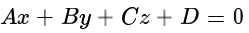即x、y、z三个参数任意两个确定，第三个参数也就确定了，也就是自由度为2，比空间维度3小1
tips：向量的乘法（a’·b’=2|a||b|cosθ），注意投影关系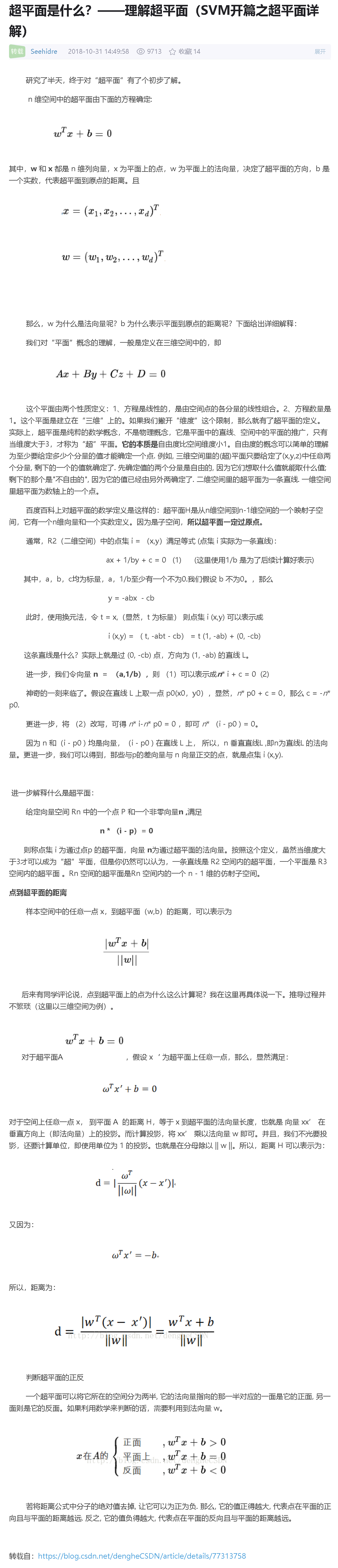展开全文• ## 【线性代数】正交投影

万次阅读 多人点赞 2014-11-16 18:07:28
注意这里我们的投影是向量的投影，几何的投影(并不一定是垂直投影的)可见度娘百科。同样的，我们从简单的二维投影来开始讨论。  1、二维投影 上图表示的是，向量b在向量a上的投影。显然有如下表达式： 其中，P...
       我们在初中就应该学过投影，那么什么是投影呢？形象点说，就是将你需要投影的东西上的每一点向你要投影的平面作垂线，垂线与平面的交点的集合就是你的投影。注意这里我们的投影是向量的投影，几何的投影(并不一定是垂直投影的)可见度娘百科。同样的，我们从简单的二维投影来开始讨论。
1、二维投影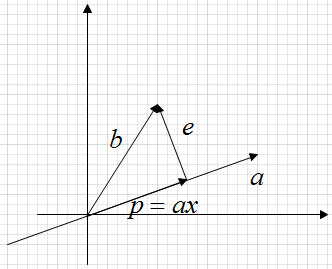上图表示的是，向量b在向量a上的投影。显然有如下表达式：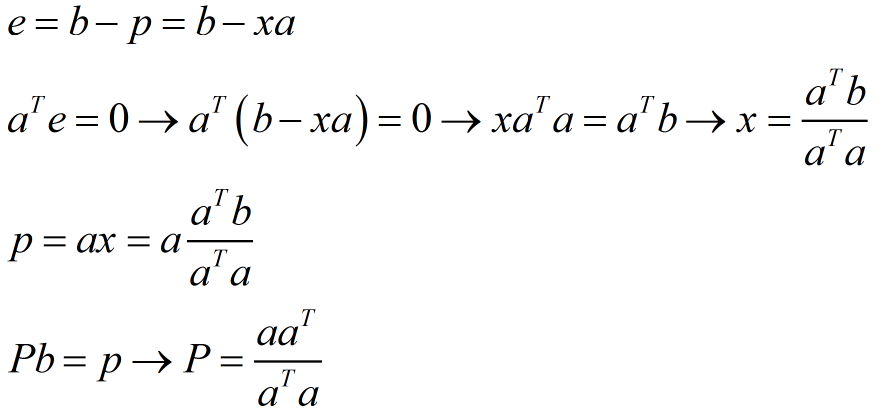其中，P为投影矩阵，由P的表达式可以看出，它具有如下性质：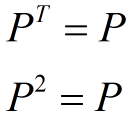2、三维投影
三维投影，就是将一个向量投影到一个平面上。同上面一样，假设是将b向量投影到平面上的p向量，则有表达式：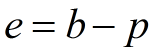e是垂直与平面的向量。由于p向量在平面上，则p向量可以由该平面的2个线性无关向量(正如，在xy平面的任何向量都可以由x轴，y轴表示)表示：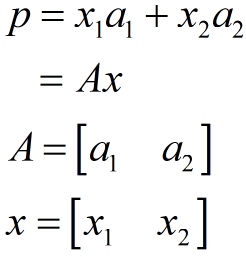由于e垂直平面，则e向量垂直与平面中的任意向量，则有：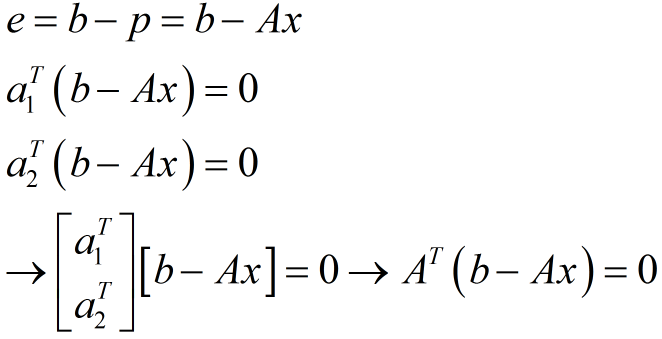将上式化简求得x：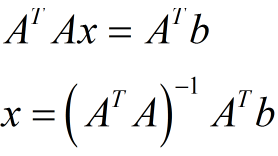又因为p=Ax，Pb=p，则得到投影矩阵为：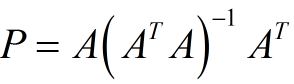由P的表达式可以看出，它具有如下性质：上面的投影矩阵是通式，当投影在一维情况时，A即为直线上的任意一个向量a,投影矩阵为：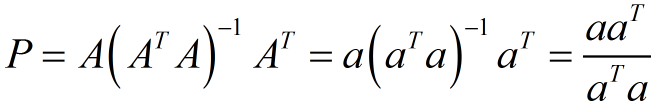注意：一个数值的逆是它的倒数。

3、举例说明
下面以一个实例来说明：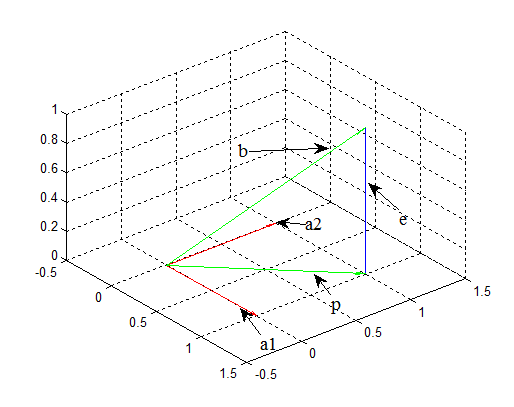如上图，假设我们要将向量b投影到水平面上，其投影为p，a1,a2为水平面的两个线性无关向量，它们的参数分别为：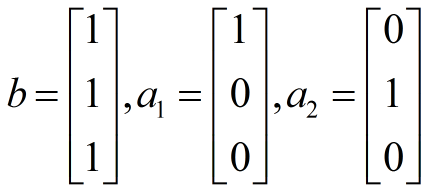那么A=[a1 a2]即：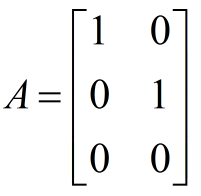由上面我们求得的通式，可得投影矩阵P: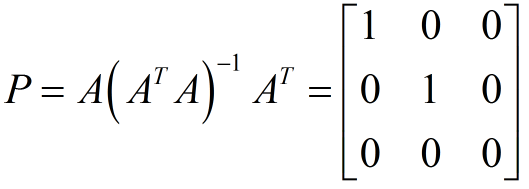知道投影矩阵P后，我们可以得到b在水平面上的投影p为：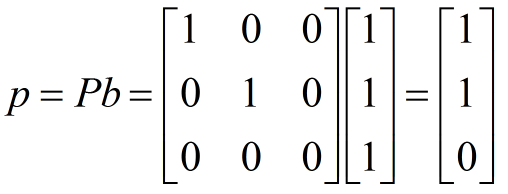显然，p与我们图中所示的结果相同。这里我们是以三维情况进行举例的，更高维情况，我们无法用图像来描述，但是通式也是成立的。

三维图的matlab程序如下：

clear all
clc

a1=[1 0 0];
a2=[0 1 0];
b=[1 1 1];
p=[1 1 0];
e=b-p;
quiver3(0,0,0,a1(1),a1(2),a1(3),1,'color','r')
hold on
quiver3(0,0,0,a2(1),a2(2),a2(3),1,'color','r')
hold on
quiver3(0,0,0,b(1),b(2),b(3),1,'color','g')
hold on
quiver3(0,0,0,p(1),p(2),p(3),1,'color','g')
hold on
quiver3(p(1),p(2),p(3),e(1),e(2),e(3),1,'color','b')原文：http://blog.csdn.net/tengweitw/article/details/41174555
作者：nineheadedbird


展开全文线性代数
• ## 正交投影

千次阅读 2017-07-22 12:41:37
注意这里我们的投影是向量的投影，几何的投影(并不一定是垂直投影的)可见度娘百科。同样的，我们从简单的二维投影来开始讨论。  1、二维投影 上图表示的是，向量b在向量a上的投影。显然有如下表达式： ...
我们在初中就应该学过投影，那么什么是投影呢？形象点说，就是将你需要投影的东西上的每一点向你要投影的平面作垂线，垂线与平面的交点的集合就是你的投影。注意这里我们的投影是向量的投影，几何的投影(并不一定是垂直投影的)可见度娘百科。同样的，我们从简单的二维投影来开始讨论。
1、二维投影上图表示的是，向量b在向量a上的投影。显然有如下表达式：其中，P为投影矩阵，由P的表达式可以看出，它具有如下性质：2、三维投影
三维投影，就是将一个向量投影到一个平面上。同上面一样，假设是将b向量投影到平面上的p向量，则有表达式：e是垂直与平面的向量。由于p向量在平面上，则p向量可以由该平面的2个线性无关向量(正如，在xy平面的任何向量都可以由x轴，y轴表示)表示：由于e垂直平面，则e向量垂直与平面中的任意向量，则有：将上式化简求得x：又因为p=Ax，Pb=p，则得到投影矩阵为：由P的表达式可以看出，它具有如下性质：上面的投影矩阵是通式，当投影在一维情况时，A即为直线上的任意一个向量a,投影矩阵为：注意：一个数值的逆是它的倒数。

3、举例说明
下面以一个实例来说明：如上图，假设我们要将向量b投影到水平面上，其投影为p，a1,a2为水平面的两个线性无关向量，它们的参数分别为：那么A=[a1 a2]即：由上面我们求得的通式，可得投影矩阵P:知道投影矩阵P后，我们可以得到b在水平面上的投影p为：显然，p与我们图中所示的结果相同。这里我们是以三维情况进行举例的，更高维情况，我们无法用图像来描述，但是通式也是成立的。
三维图的matlab程序如下：

clear all
clc

a1=[1 0 0];
a2=[0 1 0];
b=[1 1 1];
p=[1 1 0];
e=b-p;
quiver3(0,0,0,a1(1),a1(2),a1(3),1,'color','r')
hold on
quiver3(0,0,0,a2(1),a2(2),a2(3),1,'color','r')
hold on
quiver3(0,0,0,b(1),b(2),b(3),1,'color','g')
hold on
quiver3(0,0,0,p(1),p(2),p(3),1,'color','g')
hold on
quiver3(p(1),p(2),p(3),e(1),e(2),e(3),1,'color','b')

展开全文• 对于任意线性可分的两组点，它们在SVM的分类超平面的投影点都线性不可分的。为什么？ 极端假设。二维空间只存在两个点，那么它们的分类超平面就是两点连线中垂线，它们的投影点显然重合于中垂线与连线的交点。 ...
• 考虑一个二维平面，w和x都以原点为起点的向量，x在w上的投影长度可以写作: |x||w|cosθ，一个定值，设为b，则 |x||w|cosθ = b。也就是说，超平面描述了一个平面，从原点出发，末尾落到该平面上的向量...机器学习
• 文章目录从向量b投影向量a开始，从一维开始a^T^(b-xa)=0,这最关键式子这个矩阵非常有趣,让我们来看一下它性质需要记住三个公式，两个性质下面讲高维回头想一下，我们为什么要做投影？上图由a1，a2生成的平面...
• Support Vector Machine 要解决的问题：什么样的决策边界才最好的呢？支持向量机特征数据本身如果就很难分，怎么办呢？计算复杂度怎么样？能实际应用吗？...距离 = 向量(x-x')在平面向量(W)上的投影 ...
• 支持向量机（SVM）一个有监督机器学习算法，它可用于分类和回归分析，最主要用于分类问题中。 在这个算法中，根据特征值，构建一个n维空间（其中n即特征数量），把每个数据点投影到此空间内。 数据如何分类 ...
• 向量 $b = (2, 3, 4)$ 在 zzz 轴上和在 xyxyxy 平面的投影是什么，哪个矩阵能产生到一条线上和到一个平面的投影？ 当 bbb 被投影到 zzz 轴上时，它的投影 ppp 就是 bbb 沿着那条线的部分。当 bbb 被投影到一个...线性代数
• ## 矩阵论（七）：投影矩阵

万次阅读 多人点赞 2019-01-18 23:32:03
线性代数基础知识（上） 线性代数基础知识（下） 广义逆矩阵 ...如图在R3R^3R3空间中，一条直线lll与一个平面α\alphaα相交，向量zzzR3R^3R3中一个向量。把lll看成一束光（方向从上到下），光...线性代数 矩阵论
• 投影：在脑子里刻画一下，一个经典的题目：在三维坐标系下，求一个向量x在x-y平面的投影。这不是手到擒来嘛？把x的z轴分量去掉就行了，没错，就是这么简单，但是现在我们要把简单的事情标准化，让其普适起来。联想...信号处理
• 如图，两个不同向的向量 aaa， bbb，其中 bbb 落在 aaa 的方向上的向量 ppp 就是 bbb 在 aaa 上的投影，其实就是构成一个直角，这跟我们生活中的理解一样的，从图中，我们有以下的定义和结论： 向量 ppp ，它...线性代数
• 向量的引进更重要的第二类曲线积分的引进正是物理学中做功的问题。   向量及其线性运算 模，方向角（很重要），投影（直接运用公式）； 数量积，向量积和混合积   曲面及其方程（双变量） 空间曲线及其...
• 支持向量机（SVM）一个有监督机器学习算法，它可用于分类和回归分析，最主要用在分类问题中。 在这个算法中，根据特征值，构建一个n维空间（其中n即使特征数量）把每个数据点投影到此空间内。 2、数据如何分类...机器学习 数据处理
• github上项目，跟着一起学习 ... 1、什么是SVM 有监督 可用于分类和回归，主要用于分类 根据特征值，构建n维空间，数据点投影到该空间 ...最大化一个距离，这个距离到超平面最近距离 4、线性和非线性...
• 算法原理：假设带有孔的空间曲面，其外轮廓顺时针转动，内轮廓逆时针转动，那么沿着...2.将被判断的点投影到与法向垂直的面上，该平面通过曲线上引出法向的那一点，并连接投影点与法向量的原点，作为投影向量。 3...
• 感谢陈宇航同学文章，http://blog.renren.com/share/223053791/15536933699/0 从“几何”角度来看待傅里叶...1. 什么是投影 我们先来复习什么是投影吧。考虑一个简单二维平面的例子。如下图所示，给定两个向量 u数学 傅里叶级数
• 往曲面的切平面投影，得到向量就是测地曲率向量，这个向量的大小就是测地曲率。所以从定义上看，测地曲率刻画了曲线在曲面内蕴的弯曲程度，而法曲率刻画了曲线在嵌入空间的弯曲程度。比如一张平面上的直线的测地...几何
• 最小二乘法与矩阵的...二维平面向量b在向量a上的投影： 现在我们假设投影点是向量上的一点p，可以规定p⃗ \vec{p}=x⋅\cdota⃗ \vec{a}（x某个数）。定义e⃗ \vec{e}=b⃗ \vec{b}-p⃗ \vec{p}，称e⃗ \最小二乘法
• PLA停止的条件：找到一条“best”的直线可以把圆圈和叉叉完美的分开。 这就要求资料data线性可分的...上面的公式含义：两个向量的内积几何意义，一个向量在另一个向量上投影的长度。所以就是平面上的某一个点x到
• 1.起源——欧拉公式 ，i是什么，欧拉公式在复平面的意义。eix其实构成了完备标准正交基。 i代表了旋转。 欧拉恒等式在数学中严谨可以用泰勒公式推导得出，由eix和sinx cosx泰勒展开可得。 eix可以理解为一个...数字信号处理 欧拉公式 拉普拉斯变换
• 标量● 向量（矢量）● 矩阵● 张量五、范数六、行列式七、常见矩阵类型八、矩阵计算8.1 矩阵加减法8.2 数乘（叉乘）8.3 点乘8.4 矩阵相乘九、矩阵求逆十、内积与投影十一、余弦相似度十二、欧式距离 ...
• 所以我想我知道正交投影原理了，所有点沿着与相机朝向向量平行打到视口所在平面上,如果区域小，就按比例缩放到视口那样 如果区域比视口大话，但他们在一个平面上，可以缩放到视口上，也就是什么呢，正交投影...
• 最大重构性：找到一个超平面使得样本点在这个超平面的投影尽量分开。 PCA也可以看作逐一筛选方差最大方向；对协方差矩阵XX^T特征分解，取最大特征值及其特征向量；在去掉该特征值以及特征向量后，继续取最大特征值...